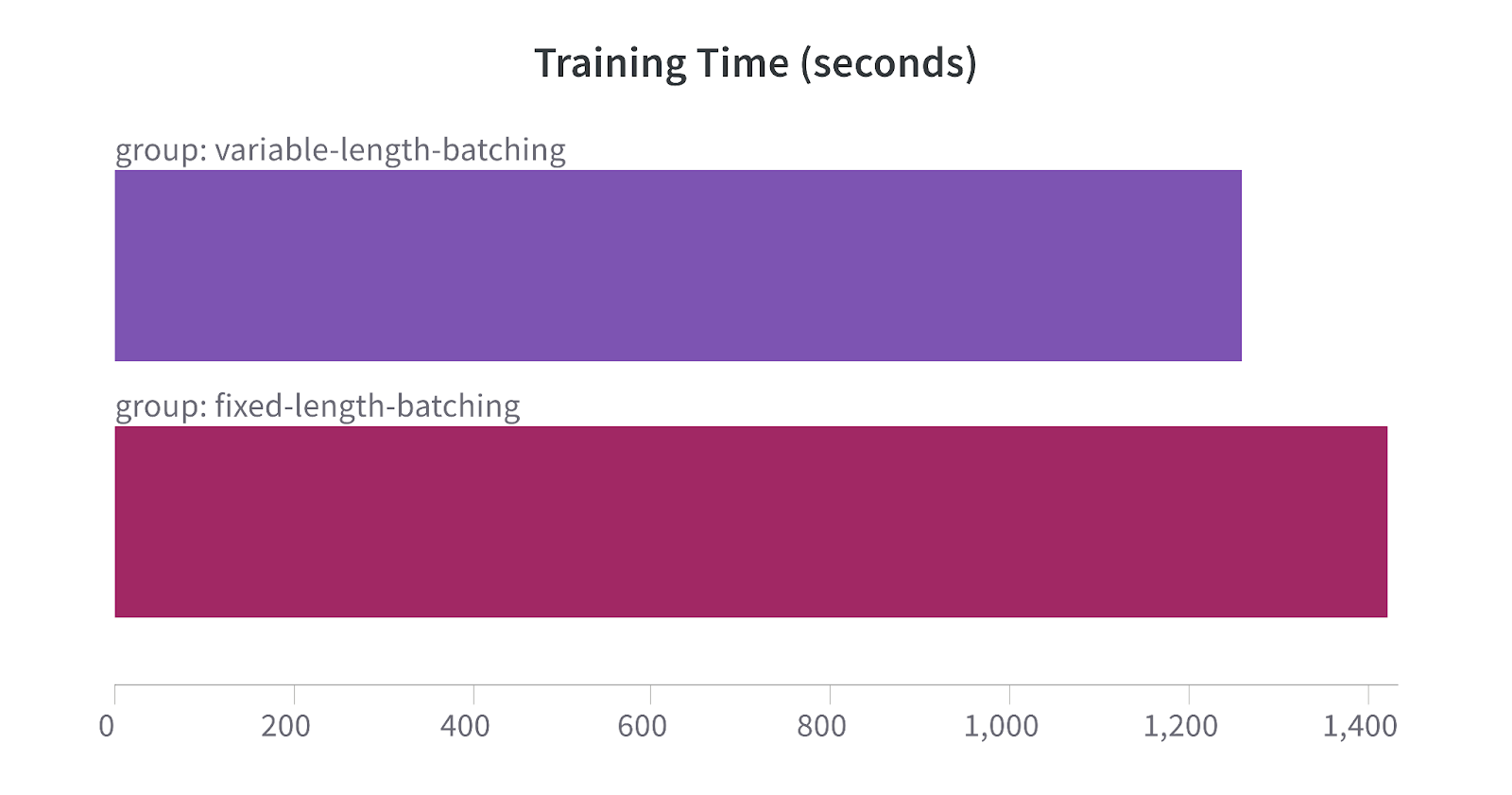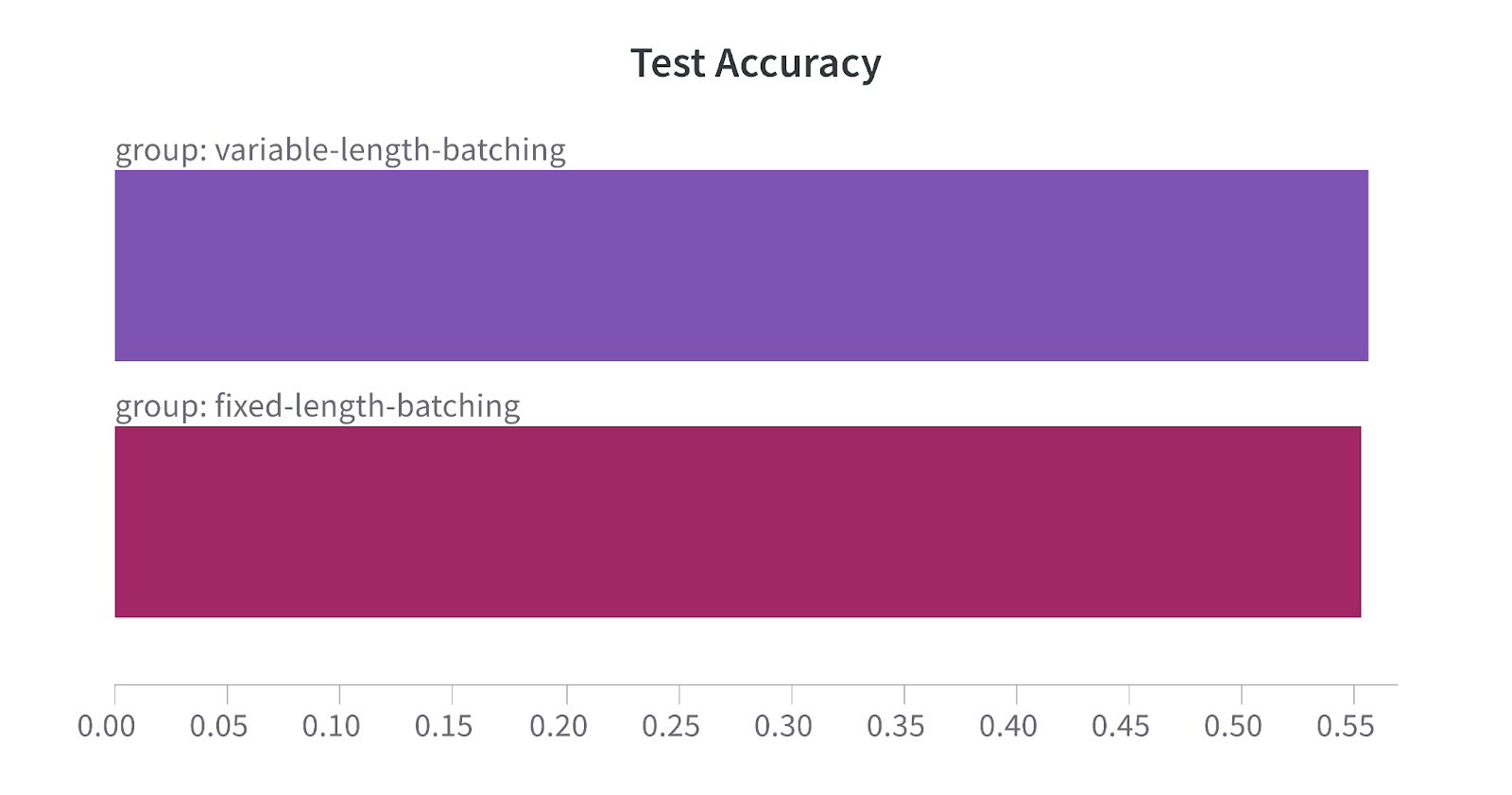# Variable-Length Sequences in TensorFlow Part 2: Training a Simple BERT Model

In this article we demonstrate how to use a BERT model with variable-length text data while minimizing training time.

In Part 1 of this series, we discussed a strategy to efficiently handle variable-length text data for simpler models. In this article, we will discuss the changes we need to make to our data pipeline when dealing with more complex models such as BERT.

## General setup

As in Part 1, we’ll be using this dataset from Kaggle which provides a text classification problem. Given a movie description of several sentences, our trained model should predict the genre out of 27 possible choices.

In Part 1, we already tokenized and encoded our descriptions and genres to prepare them for further processing.

Next, we’ll convert the descriptions and their labels (i.e. their genres) to TensorFlow Records (TFRecords). Especially when using TensorFlow, serializing our data in this way means that we only need to apply preprocessing once during serialization rather than applying it each time data is loaded on demand.

Text preprocessing typically includes stemming and lemmatization, tokenization, stopword and punctuation removal, and so on. You can refer to this tutorial for a refresher on TFRecords.

In our case, we’ll be using a Sentence BERT model for encoding the movie descriptions but the discussed strategies will work with other sequence models as well. We have two preprocessing options here:

• Treat each description as a single sentence and tokenize it with an appropriate tokenizer.
• Split each description into sentences and then tokenize them.

In this article, we’ll cover the first option and the latter option will be covered in Part 3 of this series. Ultimately, we’ll take the tokenized descriptions and their labels and serialize them into multiple TFRecord shards.

Full-fledged versions of the code shown in this article are available on GitHub.

## Tokenizing the descriptions

The movie descriptions will be stored as byte features and their labels will be stored as integer features inside the TFRecords.

The BERT tokenizer we’ll be using produces a RaggedTensor. For example, consider the following movie description:

“Alan Bennett and director Nicholas Hytner discuss and dissect the process they went through to produce the final version of The Habit of Art, the critically acclaimed play in which a group of actors rehearse a play about W.H. Auden and Benjamin Britten.

Given this description, the tokenizer will output the below:

``````# `tf` is aliased as `import tensorflow as tf`
<tf.RaggedTensor [[, , , , , [1044, 22123, 3678], , , [4487, 11393, 6593], , , , , , , , , , , , , , , , , , , , , , , , , , , [2128, 26560, 3366], , , , , , , , [8740, 4181], , , , ]]>``````

However, this RaggedTensor cannot be directly serialized and it must first be flattened. We also need to store the row splitting information so that the tensor can be constructed from the ragged dimensions when it is deserialized.

The flattened RaggedTensor and row splits are serialized as integer features stored in a list (of type `tf.train.Int64List`). We can see this in practice with the following code:

``````def _ints_feature(int_input: int) -> tf.train.Feature:
"""Encoded given data as an integer feature."""
int64_list = tf.train.Int64List(value=int_input)
return tf.train.Feature(int64_list=int64_list)

def ragged_feature(
ragged_input: tf.RaggedTensor, name: str
) -> Dict[str, tf.train.Feature]:
"""Returns a dictionary to represent a single ragged tensor as int64 features."""
int64_components = {f"{name}_values": _ints_feature(ragged_input.flat_values)}

# Collecting boundary information for the ragged dimensions.
for i, d in enumerate(ragged_input.nested_row_splits):
int64_components[f"{name}_splits_{i}"] = _ints_feature(d)
return int64_components``````

To create an example inside a single TFRecord shard, we first need to create a dictionary mapping between the feature names and their values. In our case, we structured it like so:

``````feature = {
"summary": _bytes_feature(description),
"summary_tokens_len": _ints_feature([description_len]),
"label": _ints_feature([label]),
}
feature.update(ragged_feature(description_tokens, "summary_tokens"))``````

`summary` is the movie description. `summary_tokens` contains the tokenized output of a single description as shown above and `summary_tokens_len` denotes the length of the description. The full code containing these utilities can be found here.

We are also storing `summary_tokens_len` so that we can derive the maximum sequence length for a given batch. Instead of padding our inputs to the maximum sequence length allowed by BERT (512) we’ll pad with respect to the maximum sequence of a given batch.

Later in the article, we’ll show the advantage of this method over padding sequences with a global sequence length. You can also refer to this article to know its benefits beforehand.

Lastly, we simply write the TFRecord shards with the above-mentioned structure.

## Parsing the TFRecords and preparing BERT inputs

The serialized TFRecords must be parsed before being used as inputs to our BERT model, and a feature description is needed for parsing the TFRecords — ours look like so:

``````feature_descriptions = {
"summary": tf.io.FixedLenFeature([], dtype=tf.string),
"summary_tokens": tf.io.RaggedFeature(
value_key="summary_tokens_values",
dtype=tf.int64,
partitions=[
tf.io.RaggedFeature.RowSplits("summary_tokens_splits_0"),
tf.io.RaggedFeature.RowSplits("summary_tokens_splits_1"),
],
),
"summary_tokens_len": tf.io.FixedLenFeature(, dtype=tf.int64),
"label": tf.io.FixedLenFeature(, dtype=tf.int64),
}``````

The main thing to note is how we parse the ragged features. We are asking `tf.io.RaggedFeature` to look for `summary_tokens_values` in the serialized TFRecord string where the reconstruction information (the row splits) is provided in the `partition` argument.

After the ragged feature is reconstructed into its original form, it’s loaded into `summary_tokens`. ​​For a visual understanding of how encoding of ragged tensors works in TensorFlow please refer to this guide.

We can read a single TFRecord shard with this description like so:

``````example = tf.data.TFRecordDataset("demo.tfrecord")
example = tf.io.parse_single_example(example, feature_description)``````

Our final dataset preparation function looks like the below:

``````def get_dataset(split, batch_size, shuffle):
"""Prepares tf.data.Dataset objects from TFRecords."""
ds = tf.data.Dataset.list_files(f"{TFRECORDS_DIR}/{split}-*.tfrecord")
ds = tf.data.TFRecordDataset(ds)
if shuffle:
ds = ds.shuffle(batch_size * 10)
ds = ds.batch(batch_size)
ds = ds.map(preprocess_batch)
return ds``````

The `read_example()` function first parses a given TFRecord with respect to the feature description, then casts the tokens from `int64` to `int32` (which is the input expected by `bert_input_packer`):

``````def read_example(example):
"""Parses a single TFRecord file."""
features = tf.io.parse_single_example(example, feature_descriptions)
features["summary_tokens"] = tf.cast(
features["summary_tokens"].with_row_splits_dtype(tf.int64), tf.int32
)
return features``````

The `preprocess_batch()` function first calculates the maximum sequence length for the given batch and then prepares the batch for our BERT encoder:

``````# `hub` is aliased as `import tensorflow_hub as hub`
@set_text_preprocessor(
preprocessor_path="https://tfhub.dev/tensorflow/bert_en_uncased_preprocess/3"
)
def preprocess_batch(batch):
"""Batch processing utility."""
text_tokens_max_len = tf.cast(
tf.math.reduce_max(batch["summary_tokens_len"]), dtype=tf.int32,
)

# Generating inputs for the BERT model.
bert_input_packer = hub.KerasLayer(
preprocess_batch.preprocessor.bert_pack_inputs,
arguments={"seq_length": tf.minimum(text_tokens_max_len + 2, BERT_MAX_SEQLEN)},
)
bert_packed_text = bert_input_packer(
[tf.squeeze(batch.pop("summary_tokens"), axis=1)]
)

labels = batch.pop("label")
return bert_packed_text, labels``````

`set_text_preprocessor()` is a decorator that loads a given preprocessor module from TensorFlow Hub and sets its tokenizer as that of the provided function:

``````def set_text_preprocessor(preprocessor_path: str) -> Callable:
""" Decorator to set the desired preprocessor for a
function from a TensorFlow Hub URL.
Arguments:
preprocessor_path {str} -- URL of the TF-Hub preprocessor.

Returns:
Callable -- A function with the `preprocessor` attribute set.
"""
def decoration(func: Callable):

# Setting an attribute called `preprocessor` to
# the passed function
func.preprocessor = preprocessor
return func
return decoration``````

Instead of initializing the BERT packer each time a batch is processed, we can also write a custom function for implementing the packing utility. This reduces the compute and memory overhead associated with each batch, which further reduces the model training time. Our implementation is available in this notebook.

If we analyze the maximum sequence lengths of different batches we get the following plot:

As can be seen, many batches have a maximum sequence length shorter than 512. As discussed in Part 1, a fixed 512 maximum sequence length would therefore result in significant compute waste.

We acknowledge that padding with respect to the batch-wise maximum sequence length complicates the implementation but it also comes with significant performance improvements as shown in this article. Note that if this strategy does not provide a performance boost as expected it’s better to use a global sequence length (shown later in the article).

## Model training

With our data now preprocessed and encoded, we again choose to use a very simple classification model for demonstrating the advantage of variable length batching of text sequences. The model is defined as:

``````def genre_classifier(
encoder_path: str,
input_features: List[str],
train_encoder: bool,
proj_dim: int,
num_labels: int,
):
"""Creates a simple classification model."""
text_encoder = hub.KerasLayer(encoder_path)
text_encoder.trainable = train_encoder

inputs = {
feature_name: tf.keras.Input(shape=(None,), dtype=tf.int32, name=feature_name)
for feature_name in input_features
}

text_encodings = text_encoder(inputs)
projections = tf.keras.layers.Dense(proj_dim, activation="relu")(
text_encodings["pooled_output"]
)
probs = tf.keras.layers.Dense(num_labels, activation="softmax")(projections)
return tf.keras.Model(inputs=inputs, outputs=probs)``````

We initialize the model in the following way:

``````tfhub_model_uri = "https://tfhub.dev/tensorflow/bert_en_uncased_L-12_H-768_A-12/4"
proj_dim = 128
num_labels = 27

model = genre_classifier(tfhub_model_uri, bert_inputs, False, proj_dim, num_labels)``````

We train the model ten times using the Adam optimizer on the cross-entropy loss and report the average training time and test set accuracy.

The full code of model training including TFRecord parsing can be found in this notebook.

## Performance

### Training TimeFigure 2: Model training timings with variable (top) and fixed-length (bottom) sequences.

The average training time for the model when using fixed-length batches of tokens is 1423 seconds while training with variable length batches is 1260 seconds which is an 11% improvement.

### AccuracyFigure 3: Model test accuracies with variable (top) and fixed-length (bottom) sequences.

The variable-length and fixed-length batching strategies yield test accuracies of 55.59% and 55.31% respectively.

## Conclusion

While the training time difference between the two batching strategies is not as drastic as the ones reported in Part 1 using a much simpler model, the relationship still holds for a BERT-based model as the variable-length batching is around 163 seconds faster while obtaining very similar results on the test set.

In the next and final part of this series, we’ll consider the descriptions to be lists of sentences rather than single entities to test if the same lessons can be applied.